## Cylindrical WedgeThe solid cut from a Cylinder by a tilted Plane passing through a Diameter of the base. It is also called a Cylindrical Hoof. Let the height of the wedge beand the radius of the Cylinder from which it is cut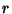. Then plugging the points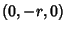,, andinto the 3-point equation for a Plane gives the equation for the plane as(1)

Combining with the equation of the Circle which describes the curved part remaining of the cylinder (and writing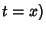then gives the parametric equations of the tongue'' of the wedge as(2)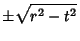(3)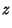(4)

for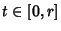. To examine the form of the tongue, it needs to be rotated into a convenient plane. This can be accomplished by first rotating the plane of the curve by 90° about the x-Axis using the Rotation Matrixand then by the Angle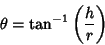(5)

above the z-Axis. The transformed plane now rests in the-plane and has parametric equations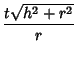(6)(7)

and is shown below.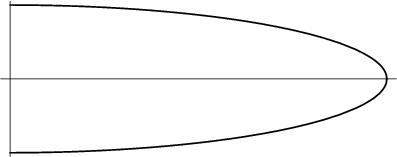The length of the tongue (measured down its middle) is obtained by plugginginto the above equation for, which becomes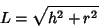(8)

(and which follows immediately from the Pythagorean Theorem). The Volume of the wedge is given by(9)